# TExES Physics/Math 7-12: Triangle Theorems & Proofs Chapter Exam

Exam Instructions:

Choose your answers to the questions and click 'Next' to see the next set of questions. You can skip questions if you would like and come back to them later with the yellow "Go To First Skipped Question" button. When you have completed the practice exam, a green submit button will appear. Click it to see your results. Good luck!

Answered 0 of 30

### Page 1

#### Question 1 1. If triangle ABD is congruent to triangle CDB, CPCTC explains which of the following statements?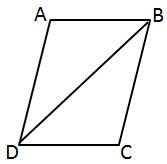#### Question 2 2. If triangle ABC is congruent to triangle ADC, what must also be true?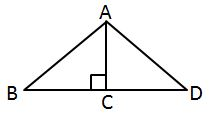#### Question 3 3. Given triangles DAR and KMR. What is the value of y?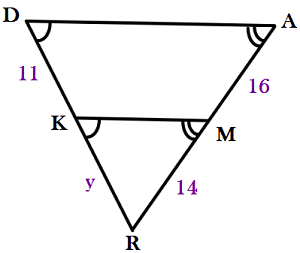#### Question 4 4. Which of the following represents the angle bisector theorem for the pictured triangle?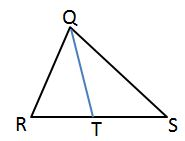#### Question 5 5. Triangle ABC is congruent to triangle XYZ. What reason can we use to explain why angle A is congruent to angle X?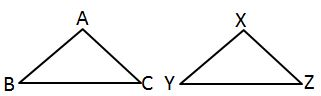### Page 2

#### Question 6 6. What additional information do you need to be given to prove that triangle PQS is congruent to triangle SRP using the HA theorem?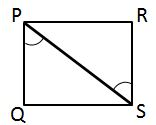#### Question 8 8. In the pictured triangles, what reason can we use to explain that angle QPR is congruent to angle SPT?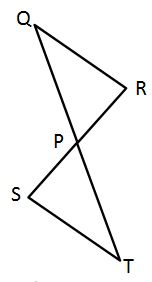#### Question 10 10. If the pictured triangles are congruent, what reason can be given?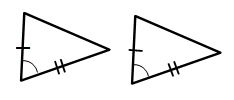### Page 3

#### Question 11 11. What additional information do you need to prove that triangle ABC is congruent to triangle DEF using the HL theorem?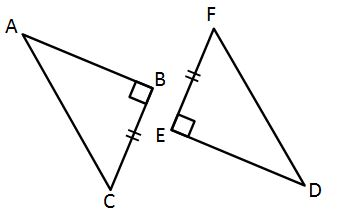#### Question 13 13. If triangle MNO is congruent to triangle PQR, CPCTC explains which of the following statements?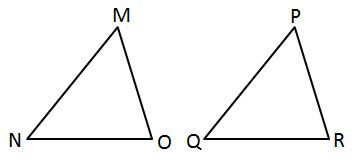#### Question 14 14. If triangle MNO is congruent to triangle STR, what must also be true?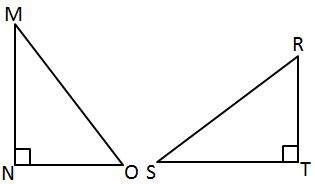#### Question 15 15. If triangle ACB is congruent to triangle YXZ, all of the following are true EXCEPT: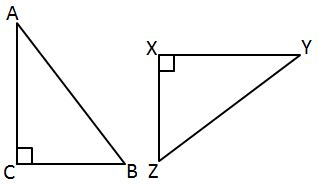### Page 4

#### Question 17 17. Which theorem or postulate can be used to establish congruence with the pictured triangles?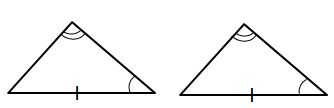#### Question 18 18. AD is a perpendicular bisector. Find the measure of AC: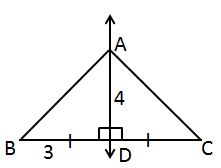#### Question 19 19. What additional information do you need to prove that triangle ACD is congruent to triangle DBA using the HL theorem?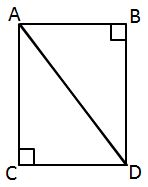#### Question 20 20. Which statement about the pictured triangle must be true?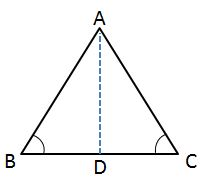### Page 5

#### Question 21 21. In the pictured triangle, AD is an angle bisector. What is the length of AC?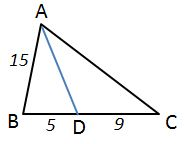#### Question 23 23. Find the measure of MN: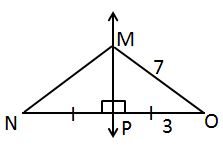#### Question 24 24. In the pictured triangle, XV is an angle bisector. What is the length of XY?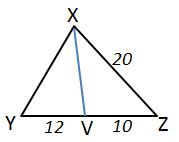### Page 6

#### Question 26 26. All of the following statements about the pictured triangle must be true, EXCEPT: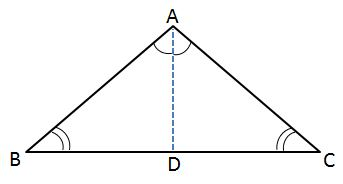#### Question 27 27. Find the measure of XZ: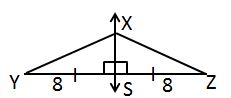#### Question 28 28. In the pictured triangle, MS is an angle bisector. What is the length of SO?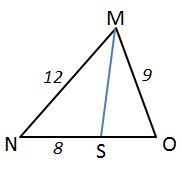#### Question 30 30. If the pictured triangles are congruent, what reason can be given?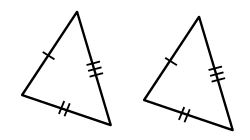#### TExES Physics/Math 7-12: Triangle Theorems & Proofs Chapter Exam Instructions

Choose your answers to the questions and click 'Next' to see the next set of questions. You can skip questions if you would like and come back to them later with the yellow "Go To First Skipped Question" button. When you have completed the practice exam, a green submit button will appear. Click it to see your results. Good luck!

Support